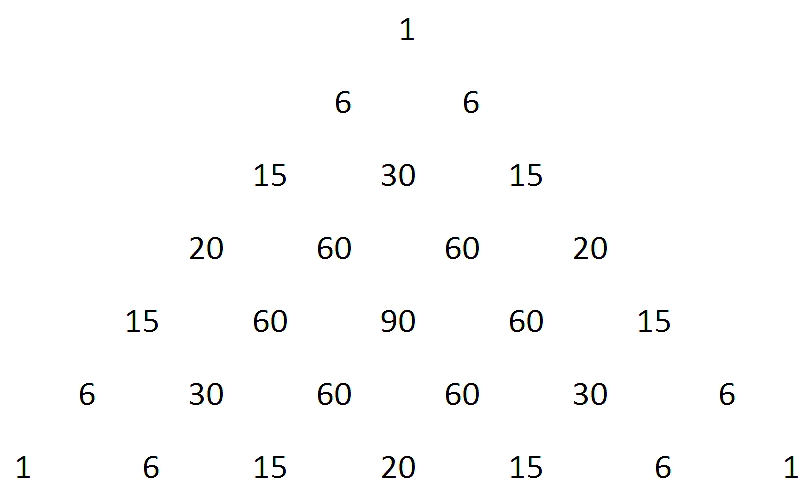## Tetrahedral Pyramids (part 2 of 3)

by

The first post in this series, Pascal’s Pyramid (part 1 of 3), explored number patterns that arise in a 3 dimensional version of Pascal’s Triangle: a pyramid with square cross sections.  Another way to do a 3D version of Pascal’s Triangle would use triangular cross sections.   If you think of building a tetrahedron by stacking oranges in a pile, each orange is in contact with (up to) three oranges that lie in the level above it.

As before, we set the top number in the pyramid to be 1, and assume that at the lower levels, each number will be the sum of the (up to) three adjacent numbers on the previous level.

Also as before, we know to expect the binomial coefficients to appear on each of the three triangular faces of our new pyramid.

In the interior, the numbers generated satisfy the recursion

f(n+1, a+1, b+1) = f(n, a, b) + f(n, a, b+1)+ f(n, a+1, b+1)

where f(0,0,0) = 1, and f(n,a,b) is understood to be zero if any of the parameters go out of range (that is, if a or b is either negative or greater than n, or if b>a).

The resulting pyramid:

And for example, the numbers on the seventh level from the top are:Examining this triangle, we recognize an odd variation on Pascal’s triangle:  each row is a multiple of a row of the usual Pascal triangle, where the multiplier is the left-most entry in the row.  Since those terms are also terms on an outer face of the pyramid, we know they too are binomial coefficients, and we are led to conjecture that$f(n, a, b) = { n \choose a } {a \choose b}$.

As before, we can prove this result by induction on n.  The base case, when n=0, works, since${0 \choose 0} {0 \choose 0} = 1$.

If we assume (for some specific value of n)  that each$f(n, a, b) = {n \choose a} { a \choose b}$, then it follows that$f(n+1, a+1, b+1) = {n \choose a}{a \choose b} + {n \choose a}{a \choose {b+1}}+ {n \choose {a+1}}{{a+1} \choose {b+1}}$$= {n \choose a} \bigg( {a \choose b} + {a \choose {b+1}} \bigg) + {n \choose {a+1}}{{a+1}\choose {b+1}}$$= {n \choose a}{{a+1} \choose {b+1}} + {n \choose {a+1}}{{a+1} \choose {b+1}}$$= {{n+1} \choose {a+1}}{{a+1}\choose {b+1}}$

And thus we have our identity by induction on n.

This suggests a direction for exploration:  what interesting things do we know about Pascal’s Triangle, that might generalize to three dimensions?

Coming up:  Sierpinski’s Pascal Pyramid (3/3)

### 3 Responses to “Tetrahedral Pyramids (part 2 of 3)”

1.Pascal’s Pyramid (part 1 of 3) « 360 Says:

[…] up:  Tetrahedral pyramids (2/3);  Sierpinski’s Pascal Pyramid (3/3) Possibly related posts: (automatically […]

2.Badal Says:

This is really cool stuff! I think it would be interesting to make a connection with polynomials (perhaps in more than one variable).

3.Badal Says:

To follow up on my previous comment. Just as$n \choose a$ gives the coefficients in the expansion of$(1+x)^n$,
1)${n \choose a} {n \choose b}$ are coefficients in the expansion of$(1+x)^n (1+y)^n$.
2)${n \choose a} {a \choose b}$ are coefficients in the expansion of$(1+x+y)^n$.
I think this should help establish a connection with the geometry.